Accepted for publication in Computational Particle Mechanics, pp. 1-11, 2019
DOI: 10.1007/s40571-019-00278-5

Abstract

We present a numerical procedure for elastic and non linear analysis (including fracture situations) of solid materials and structures using the Discrete Element Method (DEM). It can be applied to strongly cohesive frictional materials such as concrete and rocks. The method consists on defining non-local constitutive equations at the contact interfaces between discrete particles using the information provided by the stress tensor over the neighbour particles. The method can be used with different yield surfaces and in the paper it is applied to the analysis of fracture of concrete samples. Good comparison with experimental results is obtained.

Keywords: Discrete Element Method, DEM, concrete, elasticity, non linearity, geomaterials, yield surface

1 INTRODUCTION

The Discrete Element Method (DEM) has proven to be a very useful numerical tool for the computation of granular flows [2,3,4] (the hereafter termed non-cohesive DEM) with or without coupling with fluids [5,6] or structures . These computations can include cohesive forces between particles  to model moisture, glue or other added features to the standard non-cohesive DEM. Other research lines have focused on the DEM as a method to compute the mechanics of strongly cohesive materials, like rocks, concrete or cement [9,10,19]. The approach in these cases is usually termed as 'bonded' or 'cohesive' DEM. Here the DEM can be understood as a discretization method for the continuum. The bonded DEM has also been combined with the Finite Element Method (FEM) in order to save computation time .

The ability of the DEM to reproduce multi-cracking phenomena in cohesive materials is probably one of the main reasons why the DEM is chosen when fracture mechanics is an important ingredient of the solution. However, a deep analysis of the works published usually reveals a lack of accuracy of the DEM results in the elastic regime, together with the need for calibrating the DEM parameters for each application. It is quite surprising that the Poisson's ratio and the shear modulus are seldom validated. It is however commonly accepted  that the Poisson's ratio has a strong dependency on the mesh arrangement and the ${\textstyle k_{t}/k_{n}}$ ratio , where ${\textstyle k_{n}}$ and ${\textstyle k_{t}}$ are the normal and tangential spring stiffnesses, respectively, in the spring dash-pot model that yields the forces at the contact interface between two spheres.

The difficulty of the bonded DEM to get accurate results when trying to capture simultaneously the Young's modulus (${\textstyle E}$), the Poisson's ratio (${\textstyle \nu }$) and the related shear modulus (${\textstyle G}$) derives from the fact that the bonded DEM works as a system of trusses instead of a true continuum. Usually, a good calibration of the micro parameters (${\textstyle k_{n}}$ and ${\textstyle k_{t}}$) leads to a decent capture of one or two of the elastic macro parameters (${\textstyle E}$, ${\textstyle \nu }$ and ${\textstyle G}$) for a given mesh arrangement and usually for a certain, limited, range of values . Due to these limitations, the spring dash-pot model has proven not to be good enough to capture the elastic behavior of a continuum with the DEM.

In a recent paper  we proposed a way to enrich the spring dash-pot model in such a way that the elastic properties of a continuum can be accurately captured with the DEM. The improved bonded-DEM approach is based on the definition of a non local constitutive model at each contact interface in which the force-displacement relationship at an interface of a spherical particle depends on the forces at all the interfaces shared by the particle. The good properties of the new non local bonded DEM procedure for predicting the elastic behavior of elastic continua modelled as a collection of regular and irregular distributions of spherical particles was shown. Indeed the non local bonded DEM procedure can be extended for modelling breakage and separation of particles in order to reproduce the non linear behavior of a continuum, leading to fracture and failure. In this work we propose a new criterion for breaking the bonds between spherical particles based on the stress tensor at the contact interface. The stress tensor is very commonly used to trigger cracks in continuum mechanics, like in analytic solutions or in the FEM, but has not yet been used in DEM.

The arrangement of the paper is as follows. The non-local constitutive equations between forces and displacements at a contact interface are presented first. Also we describe how the stress tensor at the contact interface can be computed. The method for predicting the onset of fracture at a contact interface is then described. The last section of the paper presents the application of the new non-local bonded DEM technique to the analysis of Uniaxial Compression Strength (UCS), a Brazilian Tensile Strength (BTS) test and a shear tests in concrete samples. Numerical results are compared with experimental data for the same tests with good agreement.

2 THE STRESS TENSOR OVER DISCRETE ELEMENTS

The stress tensor, understood here as the Cauchy stress tensor, has been widely used in the context of the DEM. It is typically used to plot the value of the stresses in certain regions of the domain when dealing with granular materials . For example, it is common to model soils with the DEM and to plot the stresses within the soil as a valuable engineering result.

The averaged stress tensor over the volume of a central spherical particle, (hereafter termed particle 0) (Figure 1), can be calculated as

 ${\boldsymbol {\sigma _{0}}}={\frac {1}{V_{0}}}\sum _{i=1}^{n_{c}}{\boldsymbol {l_{i}}}\otimes {\boldsymbol {F_{i}}}}$
(1)

In Eq.1, index 0 denotes the central particle where stresses are computed, ${\textstyle V_{0}}$ is the volume of the spherical particle, ${\textstyle n_{c}}$ is the number of contacts of the particles with its neighbours, ${\textstyle {\boldsymbol {l}}_{i}}$ is the vector connecting the center of the sphere to the ${\textstyle i}$th contact point and ${\textstyle {\boldsymbol {F_{i}}}}$ is the force vector at the ${\textstyle i}$th contact point. The contact points can account for a certain gap between adjacent particles, as explained in .

Apart from granular non-cohesive materials, the DEM has been used to model strongly cohesive materials like concrete or rocks  by means of the standard bonded DEM, which can withstand tractions at a contact interface. The packing of spheres is expected to work as an equivalent continuum and the stress tensor at the center of each particle can be calculated with Eq.1 as well.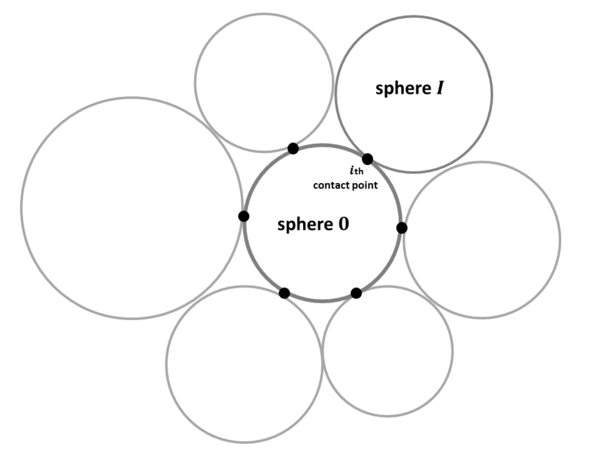Figure 1: $i$th contact point between a central sphere (0) and an adjacent sphere (I)

The idea of using the stress tensor at a particle to enrich the information used to compute the forces between bonded particles emulating a continuum was first presented Celigueta et al. . The normal force at a contact point is computed in terms of the nodal overlap and the stresses at the contact point as

 $F_{{z'}_{i}}=k_{ni}\delta _{z'i}+A_{i}\nu (\sigma _{x'i}+\sigma _{y'i})$
(2)

where ${\textstyle F_{{z'}_{i}}}$ is the force between the two particles in the normal direction ${\textstyle z'}$ (defined by the vector that joins the particle centers as shown in Figure 2), ${\textstyle A_{i}}$ is the contact area at the ${\textstyle i}$th contact interface between the two particles (particle ${\textstyle 0}$ and particle ${\textstyle I}$, see Figure 1), ${\textstyle \delta _{z'i}}$ is the overlap between the particles, ${\textstyle \nu }$ is the Poisson's ratio and ${\textstyle k_{ni}}$ is a normal stiffness parameter associated to each pair of particles given by

 $k_{ni}={\frac {A_{i}E}{L_{0i}}}$
(3)

where ${\textstyle L_{0i}}$ is the distance between the centers of the particles at the stress-free position and ${\textstyle E}$ is the Young's modulus of the continuum material .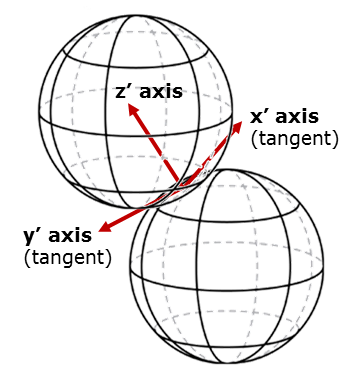Figure 2: Local axes at contact point between two spheres

In Eq.2 ${\textstyle \sigma _{x'i}}$ and ${\textstyle \sigma _{y'i}}$ are the axial stresses at the contact point in the two orthogonal directions to the normal one. They can be obtained by rotating the coordinate system for the stress tensor at the ${\textstyle i}$th contact point, ${\textstyle {\boldsymbol {\sigma }}_{i}}$, as follows (Eq.4)

 ${{\boldsymbol {\sigma }}'}_{i}={\boldsymbol {R}}_{i}^{T}{\boldsymbol {\sigma }}_{i}{\boldsymbol {R}}_{i}$
(4)

where ${\textstyle {\boldsymbol {R}}_{i}}$ is the rotation matrix between the Cartesian and the local axes of contact ${\textstyle i}$ and ${\textstyle {{\boldsymbol {\sigma }}'}_{i}}$ is the stress tensor expressed in the local coordinate system at thet contact .

The stress tensor at the ${\textstyle i}$th contact point is computed by averaging the values of the stress tensors of the contacting spheres sharing the ${\textstyle i}$th contact part (sphere 0 and sphere I) via Eq.5. This gives

 ${\boldsymbol {\sigma }}_{i}={\frac {{\boldsymbol {\sigma _{0}}}+{\boldsymbol {\sigma _{I}}}}{2}}$
(5)

Note that Eq.2 is a non-local constitutive expression that relates the normal force with the values of the stress at the particles adjacent to the central sphere.

The tangential forces at the ${\textstyle i}$th contact point are similarly computed in a non-local form as

 ${\begin{array}{c}F_{{x'}_{i}}=k_{ti}\delta _{x'i}+A_{i}G\left({\frac {\tau _{z'x',i}}{G}}-{\frac {\delta _{x'i}}{L_{i}}}\right)_{step}}\\F_{{y'}_{i}}=k_{ti}\delta _{y'i}+A_{i}G\left({\frac {\tau _{z'y',i}}{G}}-{\frac {\delta _{y'i}}{L_{i}}}\right)_{step}}\end{array}}$
(6)

where ${\textstyle \tau _{z'x',i}}$ and ${\textstyle \tau _{z'y',i}}$ are the tangential components of the local stress tensor at the ${\textstyle i}$th contact point, ${\textstyle {{\boldsymbol {\sigma }}'}_{i}}$ (Eq.4). Sub-index ${\textstyle step}$ in Eqs.6 denotes the time step at which the different terms are approximated. For explicit dynamic solution schemes, step refers to the previous time step. For implicit schemes, step refers to the current time step and the term is updated iteratively. Note that both ${\textstyle \tau _{z'x',i}}$ and ${\textstyle \tau _{z'y',i}}$ depend on the stress tensor for each particle (computed by Eq.1). Therefore, their value has to be used either from the previous time step or the previous iteration. Otherwise, the forces would never be updated according to the relative displacements. The sub-index ${\textstyle step}$ in Eq.6 avoids the substitution of ${\textstyle A_{i}G\left({\frac {\delta _{x'i}}{L_{i}}}\right)_{step}}$ by ${\textstyle k_{ti}\delta _{x'i}}$, which would cancel terms and make the expression independent from the relative displacements between the particles.

We highlight that both the normal and tangential forces make use of the averaged stress tensor ${\textstyle {\boldsymbol {\sigma }}_{i}}$ at each contact point. This increases the stencil of neighboring spheres considered for computing the forces at each contact point.

Details of the computation of the stress tensor at each particle from the normal and tangential forces, the computation of the contact areas, the necessary adjustment of the porosity of the packing and the correction of the volume and mass of the particles can be found in .

In , several other adjustments are proposed aiming at avoiding calibration of the contact parameters. However, those suggestions fall out of the scope of this work, the purpose of which is to extend the applicability of non-local bonded DEM the post-elastic regime.

3 USING THE STRESS TENSOR TO COMPUTE THE CRACK INITIATION

Yield surfaces in continuum mechanics are designed to model the behavior of a specific group of materials. For example, the Rankine yield surface  is intended for concrete or other materials whose failure is mainly tension-driven, as these materials present a much higher strength in compression than in tension. As a different example, the Von Mises yield surface is typically used for metals, giving importance to the deviatoric stresses as initiators of the non linearity.

Even if these yield surfaces are used to trigger a brittle fracture, this does not mean that the macroscopic response of the sample subject to stresses presents a brittle behavior. Actually, the post-elastic behaviour depends on the shape of the sample and the load type. Thus, for the same material properties, we can see a totally brittle response in a bending test, and a smoother non-linear graph in a UCS test.

The traditional way to detect the initiation of post-elastic behavior in a continuum is the verification of a certain yield condition expressed in terms of the stress tensor written as ${\textstyle f(\sigma _{ij})\geq 0}$ .

The novel idea presented in this paper is to use the stress tensor at a bond (computed by Eq.5) and a yield surface to trigger a crack at the contact interface between two spheres in the bonded DEM.

The way to assess if a stress tensor verifies or not the yield conditions changes with each specific constitutive model. Some examples are given in Sections 5 and 6 of this work using Rankine and Mohr-Coulomb yield surfaces. Indeed, many other examples can be found in the literature of plasticity, damage and fracture mechanics.

The proposed crack initiation criterion is independent from the specific orientation of the bond, as the stress tensor represents all the orientations in a single matrix.

With this methodology, the concept of using the stress tensor for the elastic regime presented in  is extended to the post-elastic branch, as a criterion for breaking the bond.

The next section shows the behavior of concrete samples discretized by means of the bonded DEM subject to loads, whose bond breakage is ruled by the Rankine and Mohr-Coulomb yield surfaces .

The chosen yield surfaces have been introduced in DEMpack code developed by the authors  and tested with several samples under different loads. The code has been implemented within the open source Kratos Multiphysics framework . The data preparation and visualization of results in this paper was carried out with the GiD pre- and post-processor software .

4 DESCRIPTION OF THE EXPERIMENTAL TESTS

Three different experimental tests on several concrete samples were carried out at the laboratory at the Universitat Politecnica de Catalunya (UPC) . All samples were subject to an increasing load until failure. The limit stress at which the samples analyzed for each test broke was measured and averaged. The material tested was identified as a 50 MPa concrete, with a measured Young's modulus (${\textstyle E}$) of 40 GPa (coefficient of variation of 2.5% The Poisson's ratio was not measured. The same tests were modeled with the non-local bonded DEM using the Rankine and Mohr-Coulomb yield surfaces and the DEM results were compared with the experimental values. Young's modulus of ${\textstyle E=40}$ GPa and a Poisson's ratio value of ${\textstyle \nu =0.2}$ were used in all the non-local DEM computations. The three tests are described next.

4.1 Test 1. Uniaxial Compressive Strength (UCS)

A concrete cylindrical specimen of 100 mm in diameter and 200 mm long was loaded in uniaxial compression along its symmetry axis (Figure 3).

Figure 4 shows a number of broken specimens after the tests.Figure 4: Specimens after the UCS test

The average limit stress reached by the sample was 55 MPa.

For the numerical computation with the non-local bonded DEM, a random packing of 12,000 spheres was used to model the cylinder. Two plates compressed the sample at a relative velocity of 0.05 m/s and the force on the upper plate was measured, divided by the cross section of the sample and plotted as stress vs. strain of the sample. The loading velocity used in the computation does not correspond to the loading velocity of the experiments. It was chosen as fast as possible, making sure that no elastic waves were generated. The coefficient of restitution was set to 0.0 in all runs for all tests.

4.2 Test 2. Brazilian Tensile Strength (BTS) test

A cylindrical specimen of 100 mm in diameter and 200 mm long was loaded in a biaxial stress state, generated by a diametral compression that generates a perpendicular, diametral traction (Figure 5).

Figure 6 shows a number of broken specimens after the tests.Figure 6: Specimens after the BTS test

The average limit stress reached by the sample was 4 MPa.

For the numerical computation with the DEM, a random packing of 9,000 spheres was used to model the cylinder. Two plates compressed the sample and the force on the upper plate was used to evaluate the stress at the center of the sample (Eq.7) as it was done for the experimental tests. The stress was plotted vs. the elapsed time. The relative velocity of the plates was 0.1 m/s. Again, this does not necessarily correspond to the experimental loading velocity.

 $\sigma ={\frac {f}{\pi RL}}$
(7)

In Eq.7, ${\textstyle f}$ is the measured force, ${\textstyle R}$ is the radius of the sample and ${\textstyle L}$ is the length of the sample (thickness of the slice).

4.3 Test 3. Shear Strength test

Cylindrical specimens of 150 mm of diameter and 80 mm of height were used. The samples had two parallel flat ends and two inversed tubular coaxial borings set at diameter 45 mm and 4 mm wide. The depth of the borings was 10 mm, leaving an effective shear section height of 60 mm (see Figure 7 for clarification).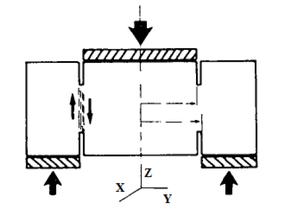Figure 7: Shear strength test scheme

The inner cylinder was pushed downwards by a piston while the outer cylinder was supported by a holed plate.

Figure 8 shows a number of broken specimens after the tests.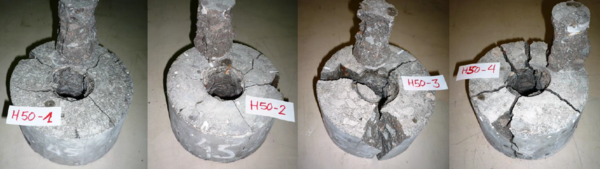Figure 8: Specimens after the Shear test

It can be observed that several types of craks were created, some around the inner cylinder of circumferencial type, some in the outer part of radial type.

For the numerical computation with the DEM, a random packing of 161,000 spheres was used to model the cylinder. The upper plate, pushing the inner part of the sample, moved downwards at a constant velocity of 0.1 m/s. The force on the upper plate was measured and plotted vs. the elapsed time.

5 DEM RESULTS WITH THE RANKINE YIELD SURFACE

5.1 Yield surface definition

The Rankine yield surface is defined as

 ${\sigma }_{1}={\sigma }_{R}$
(8)

where ${\textstyle {\sigma }_{1}}$ is the maximum principal stress and ${\textstyle {\sigma }_{R}}$ is a limit value, which can be obtained experimentally as the pure tension limit stress. We assume ${\textstyle {\sigma }_{1}\geq {\sigma }_{2}\geq {\sigma }_{3}}$, where ${\textstyle {\sigma }_{2}}$ and ${\textstyle {\sigma }_{3}}$ are the second and third principal stresses, respectively. Tractions are taken as positive. The behaviour of the material is considered elastic as long as ${\textstyle {\sigma }_{1}\leq {\sigma }_{R}}$. If ${\textstyle {\sigma }_{1}\geq {\sigma }_{R}}$ at any bond, the bond breaks. Figure 9 depicts the Rankine yield surface in the space of principal stresses.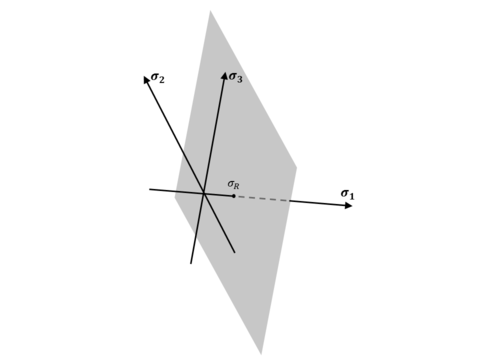Figure 9: Rankine yield surface in the space of principal stresses

5.2 Calibration of parameters

For the Rankine Yield surface, two important parameters need to be calibrated: the maximum value for a principal stress, ${\textstyle {\sigma }_{R}}$, and Coulomb's frictions parameter, ${\textstyle \mu }$. The procedure followed to calibrate those values was the following:

1. Step 1. Run Test 1 (UCS) iteratively changing the value of ${\textstyle {\sigma }_{R}}$, but keeping fixed the value of ${\textstyle \mu }$ (first computations can be run with ${\textstyle \mu =0.2}$). Coulomb's friction has little effect on the limit stress that the sample can withstand.
2. Step 2. Run Test 2 (BTS) to check that the value of ${\textstyle {\sigma }_{R}}$ yields good results and adjust the value slightly to match the experimental value.
3. Step 3. Run Test 3 (Shear) iteratively changing the value of ${\textstyle \mu }$, but keeping fixed the value of ${\textstyle {\sigma }_{R}}$ obtained after Steps 1 and 2. Once the best possible value for ${\textstyle \mu }$ is found, go to Step 1 and start the process again.

It was found that following these steps twice was enough to find a set of parameters which were useful to work with a given material.

5.3 Computational results

For all the computations, the input value of the Young's modulus was 40 MPa, and the Poisson's ratio was taken as 0.20.

After the calibration process, the limit tensile stress for the non-local DEM computations was chosen as ${\textstyle \sigma _{R}=6}$ MPa and the friction coefficient was chosen as ${\textstyle \mu =0.1}$, both between spheres and between spheres and walls. These material parameters were used for the DEM analysis of the three tests described in Section 4.

The packing of spheres used for Test 1 (UCS) contained 12K spheres and their size is detailed in the graph in Figure 10. The results of the stress-strain graph for Test 1 (UCS) are shown in Figure 11. In all cases, the horizontal lines mark the upper and lower values of the limit stress obtained experimentally. The Young's modulus which can be observed in the graph is 38 Mpa, close to the input parameter (40 MPa). Note that no calibration was needed to obtain this similarity in the elastic property, as proven in . Good agreement with the limit stress computed with the DEM is obtained. The broken sample is shown in Figure 12.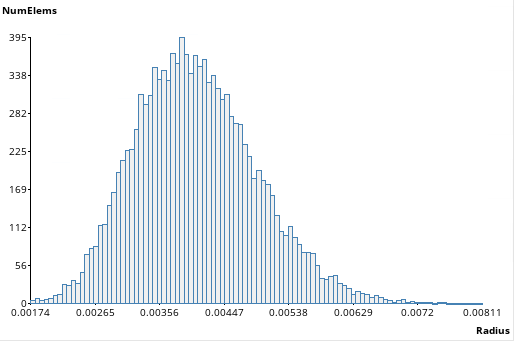Figure 10: Test 1 (UCS) sphere size distribution (in meters)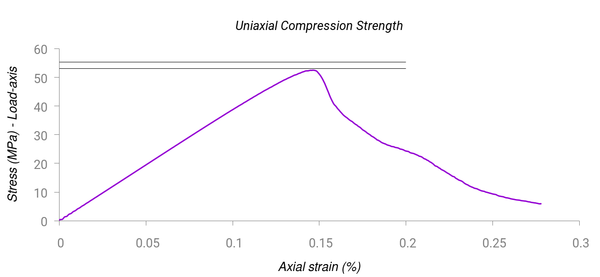Figure 11: Test 1 (UCS) with Rankine yield surface. Stress-strain curve. The horizontal lines indicate the band of experimental results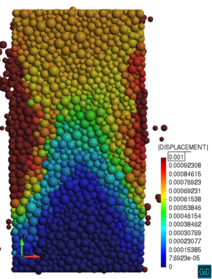Figure 12: Test 1 (UCS) with Rankine yield surface. Middle plane of a broken sample at the failure load
The packing of spheres used for Test 2 (BTS test) contained 9K spheres and their size is detailed in the graph in Figure 13. The results of the stress-time graph for Test 2 are shown in Figure 14. Good agreement with the experimental results is again obtained. The broken sample is shown in Figure 15.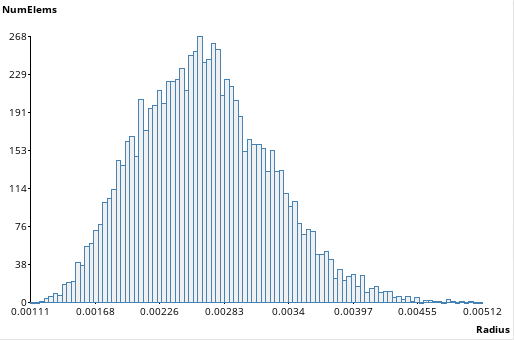Figure 13: Test 2 (BTS) sphere size distribution (in meters)Figure 14: Test 2 (BTS) with Rankine yield surface. Stress-time curve. The horizontal lines indicate the band of experimental results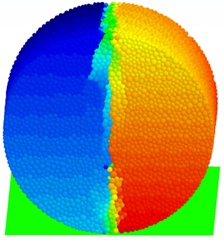Figure 15: Test 2 (BTS) with Rankine yield surface. Broken sample after the computation. Lateral displacements are plotted to visualize the cracks
The packing of spheres used for Test 3 (Shear test) contained 161K spheres and their size is detailed in the graph in Figure 16. The notches present in this geometry required spheres much smaller than in the other tests. The results of the force-time graph for the Test 3 are shown in Figure 17. The limit force obtained differs in 10% with the lower value of the experimental result. The broken sample is shown in Figure 18.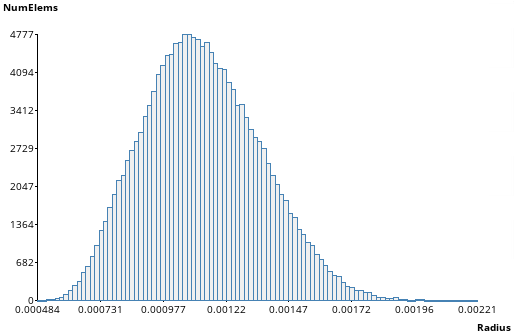Figure 16: Test 3 (Shear test) sphere size distribution (in meters)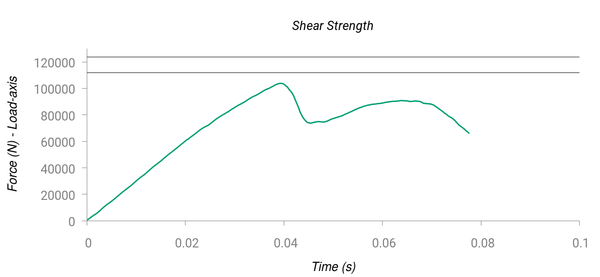Figure 17: Test 3 (Shear strength) with Rankine yield surface. Force-time curve. The horizontal lines indicate the band of experimental results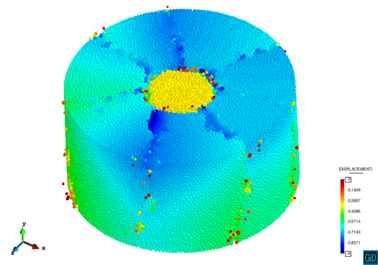Figure 18: Test 3 (Shear strength) with Rankine yield surface. Broken sample after the computation

6 MOHR-COULOMB yield surface

6.1 Yield surface definition

The Mohr-Coulomb yield surface is defined as

 ${\frac {{\sigma }_{1}-{\sigma }_{3}}{2}}=-{\frac {{\sigma }_{1}+{\sigma }_{3}}{2}}\sin(\phi )+c\cos(\phi )$
(9)

where ${\textstyle {\sigma }_{1}}$ is the maximum principal stress, ${\textstyle {\sigma }_{3}}$ is the minimum principal stress, ${\textstyle c}$ is the Mohr-Coulomb 'cohesion' stress parameter and ${\textstyle \phi }$ is the Mohr-Coulomb internal friction parameter.

6.2 Calibration of parameters

For the Rankine Yield surface, three important parameters need to be calibrated: Mohr-Coulomb strength parameters, ${\textstyle c}$ and ${\textstyle \phi }$ , and Coulomb's frictions parameter, ${\textstyle \mu }$. The procedure followed to calibrate those values was the following:

1. Step 1. Run Test 1 (UCS) iteratively changing the value of ${\textstyle c}$ and ${\textstyle \phi }$, but keeping fixed the value of ${\textstyle \mu }$ (first computations can be run with ${\textstyle \mu =0.2}$). Coulomb's friction has little effect on the limit stress that the sample can withstand.
2. Step 2. Run Test 2 (BTS) to check that the value of ${\textstyle c}$ and ${\textstyle \phi }$ yields good results and adjust the value slightly to match the experimental value. Both parameters must adjusted by the user according to the sensitivity observed.
3. Step 3. Run Test 3 (Shear) iteratively changing the value of ${\textstyle \mu }$, but keeping fixed the value of ${\textstyle c}$ and ${\textstyle \phi }$ obtained after Steps 1 and 2. Once the best possible value for ${\textstyle \mu }$ is found, go to Step 1 and start the process again.

It was found that following these steps twice was enough to find a set of parameters which were useful to work with a given material.

6.3 Computational results

The same packings of spheres used for the Rankine yield surface were used for the Mohr-Coulomb yield surface.

The values of ${\textstyle c}$ and ${\textstyle \phi }$ for all the numerical computations were calibrated to 14.5 MPa and 60 degrees, respectively. A friction coefficient of ${\textstyle \mu =0.1}$ was chosen as for the computations using the Rankine yield surface (Section 5.3). Here the calibration of the two material parameters ${\textstyle c}$ and ${\textstyle \phi }$ was more difficult than the calibration of ${\textstyle {\sigma }_{R}}$.

The results of the stress-strain graph for Test 1 (UCS), Test 2(BTS) and Test 3 (Shear Test) are depicted in Figures 19, 21 and 23, respectively. The broken samples for each case are shown in Figures 20, 22 and 24.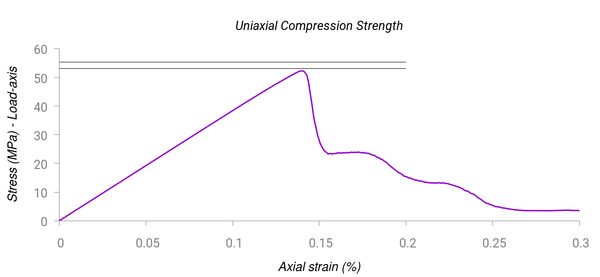Figure 19: Test 1 (UCS) with Mohr-Coulomb yield surface. Stress-strain curve. The horizontal lines indicate the band of experimental results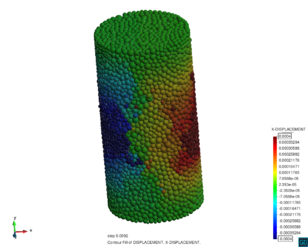Figure 20: Test 1 (UCS) with Mohr-Coulomb yield surface. External view of the broken sample after the computationFigure 21: Test 2 (BTS) with Mohr-Coulomb yield surface. Stress-time curve. The horizontal lines indicate the band of experimental results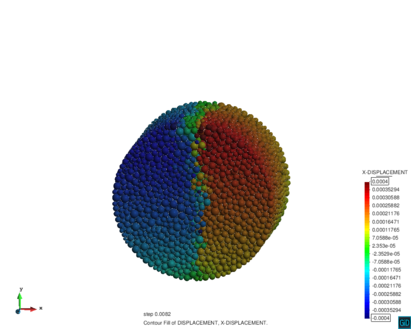Figure 22: Test 2 (BTS) with Mohr-Coulomb yield surface. Broken sample after the computation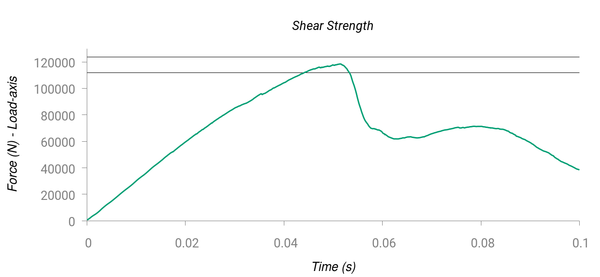Figure 23: Test 3 (Shear strength) with Mohr-Coulomb yield surface. Force-time curve. The horizontal lines indicate the band of experimental results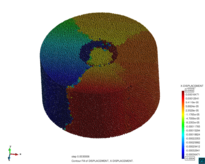Figure 24: Test 3 (Shear) with Mohr-Coulomb yield surface. Broken sample after the computation

Good agreement between the experimental values and the non-local bonded DEM results for the limit stress (UCS and BTS tests) and the limit force (Shear strength test) were obtained in all cases.

7 CONCLUSIONS

The non-local bonded DEM presented in this work can be effectively used to model the elastic range and the non-linear material behavior, at least until the solid starts collapsing, for a family of materials similar to the tested ones (concrete samples). The behavior of the material after the collapse of the sample, is left for subsequent publications. The presented approach is capable of accurately predicting the onset and initial evolution of cracks. After that, the DEM is capable of computing the displacements and rotations of any part of the solid which might get detached due to the evolution of the cracks.

The numerical examples presented in the paper have shown that the non-linear and failure behavior of concrete samples in standard laboratory tests, can be accurately predicted with the non-local bonded DEM using the the Rankine or the Mohr-Coulomb yield surfaces. The capabilities of the non-local bonded DEM, however, extend beyond the yield functions chosen in this work. Any yield surface modelling material failure that can be fed with the stress tensor can be used for non-linear analysis of solids with the non-local bonded DEM. This makes the non-local bonded DEM a powerful numerical tool for non linear analysis of a broad range of materials and structures.

The average number of bonds of each sphere (coordination number) or the size of the particles used in the computations might affect significantly the behaviour of the solid once the crack is initiated or well developed, however, this will be studied in further publications.

ACKNOWLEDGEMENTS

The authors thank Prof. Juan Miquel and Dr. Francisco Zárate for their suggestions during the development of this work.

Document informationPublished on 17/07/19

Licence: CC BY-NC-SA license

Document Score0

Views 5
Recommendations 0